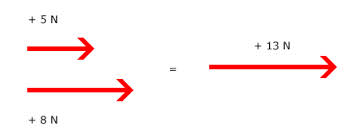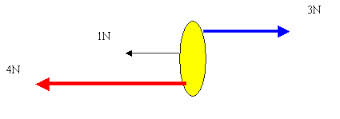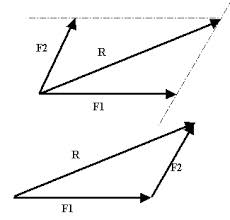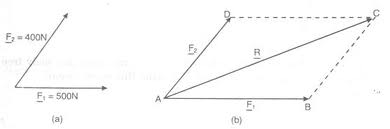## 2014-09-14

### Resultant Force I

1. Resultant force for two parallel forces

How to find the resultant force for two parallel forces?

If the forces are moving in the same direction. The resultant force is also in the direction of both forces. you have to ADD the magnitude of the two forces.If the forces are moving in opposite direction, The direction of the resultant force is the same as the larger force. Subtract the magnitude of the lesser force by the larger force.In the above example the resultant force is 1N in the direction of the larger force which is the 4N.

2. Resultant force of Two Non-Parallel Forces

When there are forces that moves in a non-parallel manner, simple calculation cannot be done to find the resultant force. What we can do is draw a scaled diagram.

Either by using the Triangle methodor the Parallelogram methodor more specificallyIn the example above, let say if we put 100N = 2 cm.

F2 = 8 cm
F1 = 10 cm

You have to measure the angle carefully using a protractor to measure the angle between the two forces. The resultant force is the R which you can measure using a ruler and convert it back to the actual magnitude (e.g. if the resultant force is 12 cm then it would convert to 600 N)! The angle is the angle between R and F1.

Can you find the resultant force for this one?#### 2 comments:

Ibphysics Tutions said...

Information given in this article is helpful for me too! This information has helped me to find Coaching For Competitive Exams
Thanks for information provided by you, it was best of all
IB Higher Level Physics | IB Standard Level Physics

Ibphysics Tutions said...

very nice and good to read, i hope that every one will like this very much thank for the posting If you would love to learn more.
IB Higher Level Physics | IB Standard Level Physics